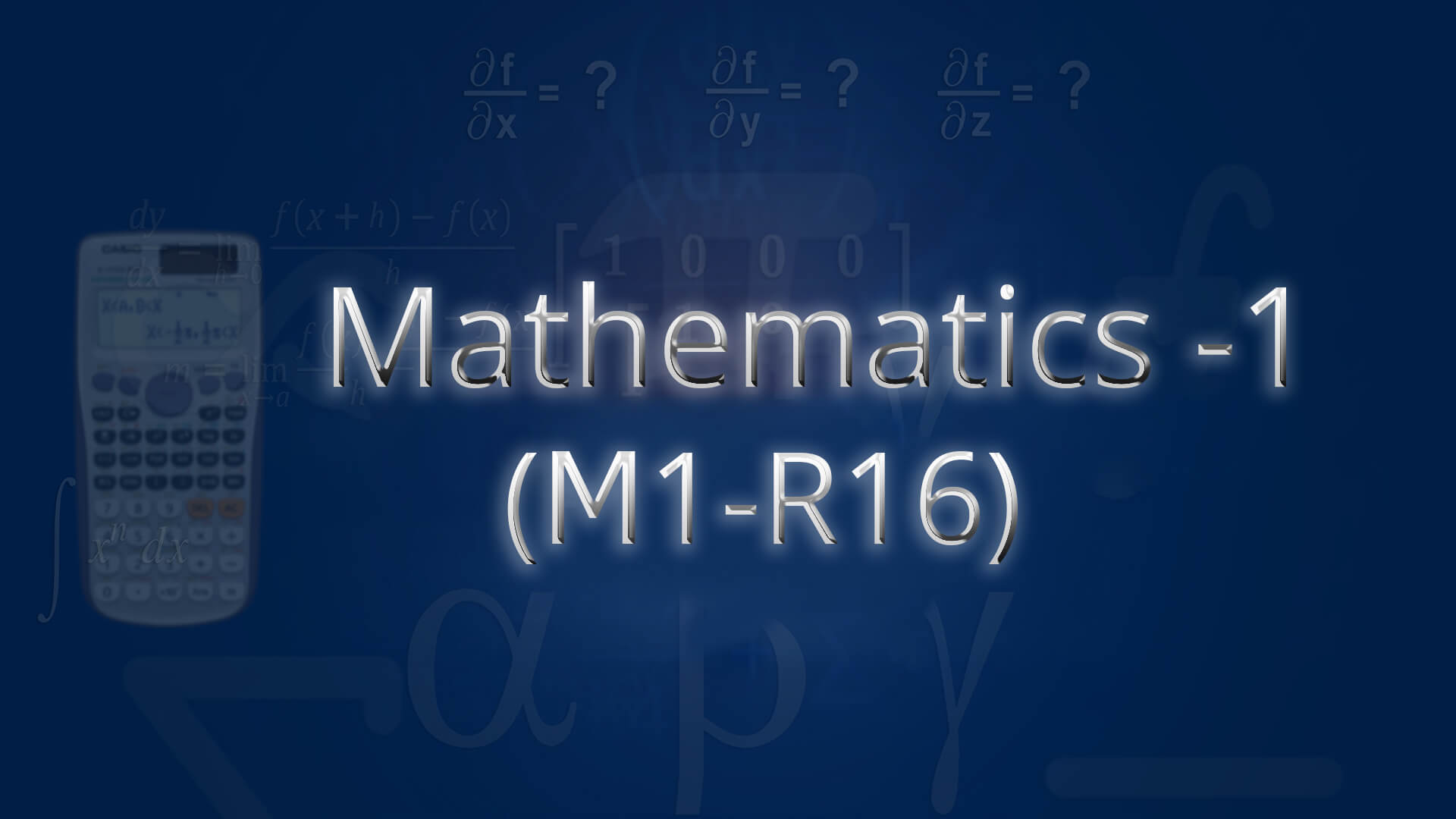## Mathematics 1(M1-R16) by Mr C Srinivas MSc ,BEd (Osmania) The course is designed to equip the students with the necessary mathematical skills and techniques that are essential for an engineering course. The skills derived from the course will help the student from a necessary base to develop analytic and design concepts. Code : M1R16B.tech1 Recommended For : B.Tech 1st Year JNTU

#### After Learning this subject,you should be able to

• Write the matrix representation of a set of linear equations and to analyze the solution of the system of equations.
• Find the Eigen values and Eigen vectors which come across under linear transformations.
• Find the extreme values of functions of two variables with/ without constraints.
• Identify whether the given first order DE is exact or not.
• solve higher order DE’s and apply them for solving some real world problems.

## Curriculum 13:19:53   units   |   5

• 3:45:28

UNIT 1
Initial Value Problems and Applications: Exact differential equations, Reducible to exact Part 1, Reducible to exact Part 2, Linear differential equations of higher order with constant coefficients - PART 1, Linear differential equations of higher order with constant coefficients - PART 2, Linear differential equations of higher order with constant coefficients - PART 3, Non homogeneous terms with RHS term of the type ax e , sin ax, cos ax 01, Non homogeneous terms with RHS term of the type ax e , sin ax, cos ax,02, Higher order differentional equations Problem 1, Higher order differentional equations Problem 2, Higher order differentional equations Problem 3, Higher order differentional equations Problem 4, Higher order differentional equations Problem 5, Higher order differentional equations Problem 6, Higher order differentional equations Problem 7&8, Higher order differentional equations Problem 9, Higher order differentional equations Problem 10, Wronskian of functions & Method of variation of parameters., Applications : Newtons law of cooling and natural growth and decay, Problems on Newtons law of cooling, Problems on natural growth and decay, Orthogonal trajectories, Problems on Orthogonal Trajectories Part 1, Problems on Orthogonal Trajectories Part 2, Problems on EDE, EDE Polar Form, Method of variation of parameters, Electrical Circuits Applications

• 2:43:20

UNIT 2
Linear Systems of Equations: Types of real matrices and complex matrices, Types of real matrices and complex matrices Part 1, Types of real matrices and complex matrices Part 2, Types of real matrices and complex matrices Part 3, Rank Of Matrix, Rank Of Matrix Problem, Echelonform Problem, Normal Form Or Canonical Form, Normalform (or) Canonical form problem, Inverse of Non-Singular matrices by Gauss-Jordan Method, Solution of system of Linear Equations, Solution of system of Homogeneous Linear Equations, Consistency and solution of linear systems(Homogeneous and Non-Homogeneous) Part 1, Consistency and solution of linear systems(Homogeneous and Non-Homogeneous) Part 2, Gauss Seidel iteration method, Gauss Elimination and Seidel iteration method Part 1 and 2

• 2:40:38

UNIT 3
Eigen values Eigen Vectors and Quadratic Forms: Eigen Values and Eigen Vectors, Eigen Values and Eigen Vectors and their properties Theorem, Eigen Values and Eigen Vectors Problems Part 1, Eigen Values and Eigen Vectors Problems Part 2, Eigen Values and Eigen Vectors Problems Part 3, Diagonalization of matrix, Diagonalization of a matric by Othogonal Reduction, Diagonalization of matrix Problem 1 and 2, Cayley-Hamilton Theorem(Without Proof), Cayley-Hamilton Theorem Problem, Finding Inverse and power of a matrix by Cayley-Hamilton Theorem, Quadratic Forms and Nature Of The Quadratic Form, Quadratic Forms and Nature of the Quadratic forms, Reduction of Quadratic Form To Canonical Form, Reduction of Quadratic form to canonical form by orthogonal transformation part-1, Reduction of Quadratic form to canonical form by orthogonal transformation part-2

• 2:05:01

UNIT 4
Partial Differentiation: Euler's Theorem, Euler's Theorem Problem 1 and 2, Total Derivative, Chain Rule of Partial Derivation, Chain Rule of Partial Derivation Problem 1, Chain Rule of Partial Derivation Problem 2, Chain Rule of Partial Derivation Problem 3, Jacobian Determinant, Jacobian Problem 1 and 2, Functional Dependence and Independence, Maxima and minima of functions of 1 , 2 variables, Lagranges Method, Max and min of functions of 2 , 3 variables using method of Lagrange Multipliers Part 1, Max and min of functions of 2 ,3 variables using method of Lagrange Multipliers Part 2&3, Taylor's Series and Maclaurin's Series, Taylor's Series and Maclaurin's Series Part 1, Taylor's Series and Maclaurin's Series Part 2

• 2:05:26

UNIT 5
First Order Partial Differential Equations: Formation of partial differential equations by elimination of arbitrary constants,functions, Formation Of P.D.E By Eliminating arbitrary Constants - Problem 1 and 2, Formation Of P.D.E By Eliminating arbitrary Constants - Problem 3, Formation of PDE By arbitrary functions - Problem 1 and 2, Formation of PDE By arbitrary functions - Problem 3 and 4, Lagranges method to solve the first order linear equations, Lagranges Linear Equation Part 1, Lagranges Linear Equation Part 2, Solutions Of Non Linear Of PDA, The standard type methods to solve the non linear equations Part 1, The standard type methods to solve the non linear equations Part 2, The standard type methods to solve the non linear equations Part 3, Standard Form II Problem 1 and 2, Standard Form III and IV, Standard Form V &VI - Problem 1 and 2